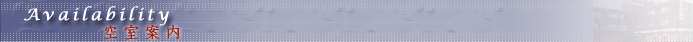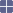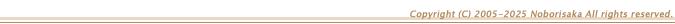> ビジネスホテル河口湖 > 空室状況シングルルーム（室数：全２１室） >> 予約申し込み1月の空室状況
1
×
2
×
3
×
4
×
5
×
6
×
7
×
8
×
9
×
10
×
11
×
12
×
13
×
14
×
15
×
16
×
17
×
18
×
19
×
20
×
21
×
22
×
23
×
24
×
25
×
26
×
27
×
28
×
29
×
30
×
31
×
2月の空室状況
1
×
2
×
3
×
4
×
5
×
6
×
7
×
8
×
9
×
10
×
11
×
12
×
13
×
14
×
15
×
16
×
17
×
18
×
19
×
20
×
21
×
22
×
23
×
24
×
25
×
26
×
27
×
28
×
3月の空室状況
1
×
2
×
3
×
4
×
5
×
6
×
7
×
8
×
9
×
10
×
11
×
12
×
13
×
14
×
15
×
16
×
17
×
18
×
19
×
20
×
21
×
22
×
23
×
24
×
25
×
26
×
27
×
28
×
29
×
30
×
31
×ツインルーム（室数：全５室） >> 予約申し込み1月の空室状況
1
×
2
×
3
×
4
×
5
×
6
×
7
×
8
×
9
×
10
×
11
×
12
×
13
×
14
×
15
×
16
×
17
×
18
×
19
×
20
×
21
×
22
×
23
×
24
×
25
×
26
×
27
×
28
×
29
×
30
×
31
×
2月の空室状況
1
×
2
×
3
×
4
×
5
×
6
×
7
×
8
×
9
×
10
×
11
×
12
×
13
×
14
×
15
×
16
×
17
×
18
×
19
×
20
×
21
×
22
×
23
×
24
×
25
×
26
×
27
×
28
×
3月の空室状況
1
×
2
×
3
×
4
×
5
×
6
×
7
×
8
×
9
×
10
×
11
×
12
×
13
×
14
×
15
×
16
×
17
×
18
×
19
×
20
×
21
×
22
×
23
×
24
×
25
×
26
×
27
×
28
×
29
×
30
×
31
×デラックスツインルーム（室数：全５室） >> 予約申し込み1月の空室状況
1
×
2
×
3
×
4
×
5
×
6
×
7
×
8
×
9
×
10
×
11
×
12
×
13
×
14
×
15
×
16
×
17
×
18
×
19
×
20
×
21
×
22
×
23
×
24
×
25
×
26
×
27
×
28
×
29
×
30
×
31
×
2月の空室状況
1
×
2
×
3
×
4
×
5
×
6
×
7
×
8
×
9
×
10
×
11
×
12
×
13
×
14
×
15
×
16
×
17
×
18
×
19
×
20
×
21
×
22
×
23
×
24
×
25
×
26
×
27
×
28
×
3月の空室状況
1
×
2
×
3
×
4
×
5
×
6
×
7
×
8
×
9
×
10
×
11
×
12
×
13
×
14
×
15
×
16
×
17
×
18
×
19
×
20
×
21
×
22
×
23
×
24
×
25
×
26
×
27
×
28
×
29
×
30
×
31
×

 ○表示 空室多くあります。Web予約申し込みができます。 △表示 空室あと少しです。Web予約申し込みできますがお急ぎください。 ×表示 ほぼ満室です。Web予約申し込みできません。 直前キャンセル等で空室が生じる場合もありますので、お電話でご確認ください。 ご予約・お問い合わせ：０５５５−７２−１２２１